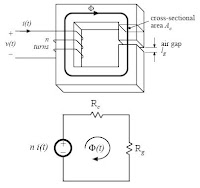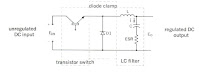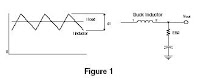# Electronic Device And Electronic Circuit

Data of electronic device , PCB Design and electronic circuitWeb basicelectronic.blogspot.com

## Thursday, March 05, 2009

### Inductor Design

Filter inductor design constraints
Objective:
Design inductor having a given inductance L,
which carries worst-case current Imax without saturating,
and which has a given winding resistance R, or,
equivalentlyIndex
- Assumed filter inductor geometry
- Constraint: maximum flux density
- Constraint: Inductance
- Constraint: Winding area
- The window utilization factor Ku
- also called the “fill factor”
- Winding resistance
- The core geometrical constant Kg
- Core geometrical constant Kg
- A step-by-step procedure

Technical Bulletin
Better efficiency, reduced size, and lower costs have combined to
make the switching regulator a viable method for converting unfiltered
DC input voltages into regulated DC outputs. This brochure describes
the switching regulator and presents design information. In particular,
MAGNETICS® Ferrite and Molypermalloy Powder cores used for
the power inductor are highlighted.

DESCRIPTIONA typical circuit consists of three parts: transistor switch, diode
clamp, and an LC filter. An unregulated DC voltage is applied to
the transistor switch which usually operates at a frequency of 1 to 50
kilohertz. When the switch is ON, the input voltage, Ein, is applied to
the LC filter, thus causing current through the inductor to increase;
excess energy is stored in the inductor and capacitor to maintain
output power during the OFF time of the switch. Regulation is
obtained by adjusting the ON time, ton, of the transistor switch, using
a feedback system from the output. The result is a regulated DC
output,

index
- COMPONENT SELECTION
- INDUCTOR DESIGN
- CORE SELECTION PROCEDURE
- DESIGN EXAMPLE
Switching Regulator Inductor Design
COILTRONICS Application Notes Magnetics
In switching regulator applications the inductor is used as
an energy storage device, when the semiconductor
switch is on the current in the inductor ramps up and
energy is stored. When the switch turns off this energy is
released into the load, the amount of energy stored is
given by;
Energy = 1/2L.I2 (Joules) (1)
Where L is the inductance in Henrys and I is the peak
value of inductor current.
The amount by which the current changes during a
switching cycle is known as the ripple current and is
defined by the equation;
V1 = L.di/dt (2)
Where V1 is the voltage across the inductor, di is the
ripple current and dt is the duration for which the voltage
is applied. From this we can see that the value of ripple
current is dependent upon the value of inductance.
Choosing the correct value of inductance is important in
order to obtain acceptable inductor and output capacitor
sizes and sufficiently low output voltage ripple.Index
- Inductor Selection for Buck Converters
- Inductor Selection for Boost Converters
- Inductor Selection for Buck-Boost Converters